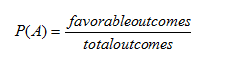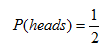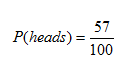Review

Probability is the ratio of the number of favorable outcome to the total number of outcomes. Probability is divided into two main categories: theoretical and experimental. Theoretical probability is the probability of what could happen. Experimental probability is the probability of what already happened during a trial. The following formula will be used to calculate probability:Example 1 What is the theoretical probability of getting a head when you flip a coin?Example 2 If a coin is flipped 100 times ends on heads 57 of those times, what is the experimental probability of flipping heads?